# Precalculus : Properties of Logarithms

## Example Questions

1 2 3 4 5 7 Next →

### Example Question #61 : Properties Of Logarithms

Expand this logarithm: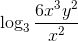Possible Answers:

None of the other answers.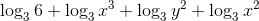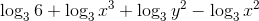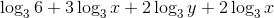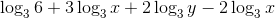Correct answer:Explanation:Use the Quotient property of Logarithms to express on a single line: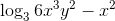Use the Product property of Logarithms to expand the two terms further: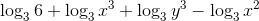Finally use the Power property of Logarithms to remove all exponents:The expression is now fully expanded.

### Example Question #62 : Properties Of Logarithms

Expand the following logarithm: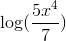Possible Answers: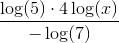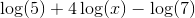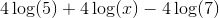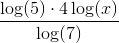Correct answer:Explanation:

Expand the following logarithm:To expand this log, we need to keep in mind 3 rules:

1) When dividing within a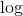, we need to subtract

2) When multiplying within a, we need to add

3) When raising to a power within a, we need to multiply by that number

These will make more sense once we start applying them.

First, let's use rule number 1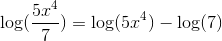Next, rule 2 sounds good.Finally, use rule 3 to finish up!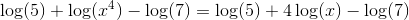Making our answer### Example Question #63 : Properties Of Logarithms

Completely expand this logarithm: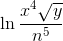Possible Answers: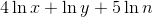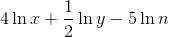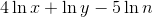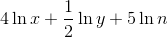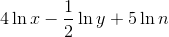Correct answer:Explanation:Quotient property: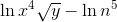Product property: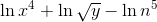Power property: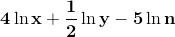### Example Question #64 : Properties Of Logarithms

Fully expand: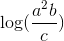Possible Answers: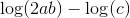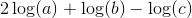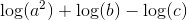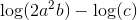Correct answer:Explanation:

In order to expand the expression, use the log rules of multiplication and division.  Anytime a variable is multiplied, the log is added.  If the variable is being divided, subtract instead.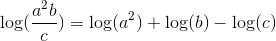When there is a power to a variable when it is inside the log, it can be pulled down in front of the log as a coefficient.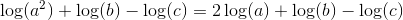The answer is:### Example Question #65 : Properties Of Logarithms

Expand the following: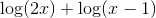Possible Answers: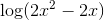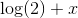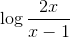Correct answer:Explanation:

To solve, simply remember that when you add logs, you multiply their insides.

Thus,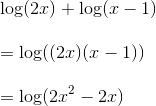### Example Question #66 : Properties Of Logarithms

Express the following in expanded form.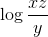Possible Answers: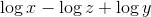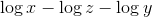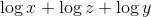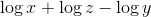Correct answer:Explanation:

To solve, simply remember that when adding logs, you multiply their insides and when subtract logs, you divide your insides. You must use this in reverse to solve. Thus,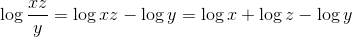### Example Question #67 : Properties Of Logarithms

Completely expand this logarithm: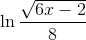Possible Answers: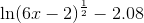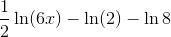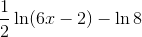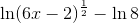The answer is not present.

Correct answer:Explanation:

We expand logarithms using the same rules that we use to condense them.

Here we will use the quotient property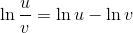and the power property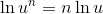Use the quotient property: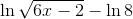Rewrite the radical:Now use the power property:### Example Question #68 : Properties Of Logarithms

Expand the logarithm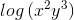Possible Answers: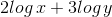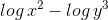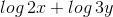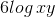Correct answer:Explanation:

In order to expand the logarithmic expression, we use the following properties

•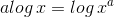•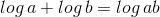As such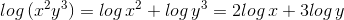### Example Question #69 : Properties Of Logarithms

Given the equation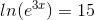, what is the value of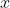? Use the inverse property to aid in solving.

Possible Answers: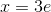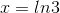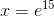Correct answer:Explanation:

The natural logarithm and natural exponent are inverses of each other.  Taking the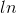of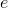will simply result in the argument of the exponent.

That is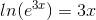Now,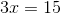, so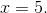1 2 3 4 5 7 Next →

### All Precalculus Resources